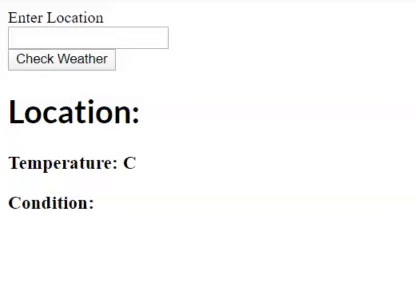# ReactJS | AJAX and API

• Last Updated : 10 May, 2020

APIs are used for fetching data from the server and using AJAX and API we call data asynchronously and show it in our HTML. You can make API requests by using browser build in fetch function or third party libraries like Axios. You can make API requests anywhere but it is totally recommended that you should make an API call in componentDidMount() life cycle method.

Reasons to use componentDidMount(): So, this can be really simple by just putting the API request in componentDidMount.

• Using componentDidMount makes sure that data won’t be loaded until after the initial render which is really important.
• Using componentDidMount make sure that the data is only fetched from client.

Prerequisites:

1. JavaScript and JSX
2. Knowledge about react state and setState
3. Knowledge about React Components and how to make them
4. React Life cycle methods

Below code example of how to use API:

• Example:
 `class MyComponent extends React.Component {``  ``constructor(props) {``    ``super``(props);``    ``this``.state = {``      ``error: ``null``,``      ``dataFetched: ``false``,``      ``data: []``    ``};``  ``}`` ` `  ``componentDidMount() {``    ``fetch(``"https://api.toptensongs.com/data"``)``      ``.then(res => res.json())``      ``.then(``        ``(response) => {``          ``this``.setState({``            ``dataFetched: ``true``,``            ``data: response.data``          ``});``        ``},``        ``(error) => {``          ``this``.setState({``            ``dataFetched: ``true``,``            ``error``          ``});``        ``}``      ``)``  ``}`` ` `  ``render() {``    ``const { error, dataFetched, data } = ``this``.state;``    ``if` `(error) {``      ``return` `
Error: {error.message}
;``    ``} ``else` `if` `(!isLoaded) {``      ``return` `
;``    ``} ``else` `{``      ``return` `(``        ``
``          ``{data.map(value => (``            ``
1. ``              ``{value.name} - {item.artist}``            ``
2. ``          ``))}``        ``
``      ``);``    ``}``  ``}``}`

So, this is a simple example in which we explained how to use API, so it is important to note that error handling is really important because if the data is not fetched it should show an error.

But here is another example if we want to load data on some actions like on click like fetching someplace’s weather then we can’t use componentDidMount() because it is only called once so for that we can use componentWillUpdate() but it was removed so, we can use a function also in place of componentWillUpdate() and make API request in a function.

• Example: So, In this example, we are going to make a really simple weather app using OpenWeatherMap API.
 `class Weather extends React.Component {`` ` `    ``constructor(props) {``        ``super``(props);`` ` `        ``this``.state = {``            ``location: ``""``,``            ``place: ``""``,``            ``temp: ``""``,``            ``weather: ``""``        ``};``    ``}`` ` `    ``render() {`` ` `        ``return` `(``            ``
``                ````                ``
``                ``
``                    ````                ``
``                ``
``                    ````                ``
``                ``
``                    ``

Location: {``this``.state.place}

``                    ``

Temperature: {``this``.state.temp} C

``                    ``

Condition: {``this``.state.weather}

``                ``
``            ``
``        ``);``    ``}`` ` `    ``changeValue = (event) => {`` ` `        ``this``.setState({``            ``location: event.target.value``        ``});``    ``}`` ` `    ``getWeather = () => {`` ` `        ``fetch(```https:``//api.openweathermap.org/data/2.5/weather?q=\${this.state.location}&units=metric&APPID=APIKEY`)``            ``.then(response => response.json())``            ``.then(data => {``                ``this``.setState({``                    ``place: data.name,``                    ``temp: data.main.temp,``                    ``weather: data.weather.main``                ``});``            ``});`` ` `    ``}``}`` ` `export ``default` `class Main extends React.Component {`` ` `    ``constructor(props) {`` ` `        ``super``(props);`` ` `        ``this``.state = {`` ` `        ``};``    ``}`` ` `    ``render() {`` ` `        ``return` `(``            ``
``                ``
``                  ``What's the Weather?``                ``
``                ``
``                ````            ``
``        ``);``    ``}``}`
• Output: So, what we did here we just moved the API request in getWeather() function and so it will only be called whenever we will make click on check weather.My Personal Notes arrow_drop_up